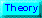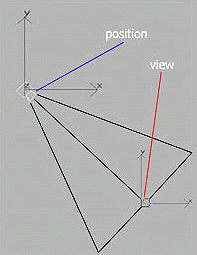# Programming - Games - OpenGL and C++

This toturial was written for me by Imran Khan (imranahmedkhan82@hotmail.com, iak1982@yahoo.com).
Copyright (c) 2004 is owned by Martin BakerUse of Camera:

In this section we will learn 'How to use Camera in Opengl' . Camera usage is the most important feature of OpenGL. As it is impossible to move in a 3D-World physically. We therefore use Camera in order to move in 3D-world.

First of all we will define a Vector Structure.

 typedef struct tVector3 { tVector3(){} // constructor tVector3 (float new_x, float new_y, float new_z) // constructor {x = new_x; y = new_y; z = new_z;} tVector3 operator+(tVector3 vVector) {return tVector3(vVector.x+x, vVector.y+y, vVector.z+z);} tVector3 operator-(tVector3 vVector) {return tVector3(x-vVector.x, y-vVector.y, z-vVector.z);} tVector3 operator*(float number) {return tVector3(x*number, y*number, z*number);} tVector3 operator/(float number) {return tVector3(x/number, y/number, z/number);} float x, y, z; }tVector3;

First we define 2 constructors, one with no arguments ( which is also called Default Constructor) and one with 3 arguments. As we are in 3D , we will use 3 coordinates (x,y,z). we assign the value of the arguments (new_x,new_y,new_z) to its local variable(x,y,z).

Next we Overload ( + , - , * , / ) Operators.So that we can easily add,subtract,multiply,divide Vectors. We will use this structure in order to move the camera.

Now declare 3 variable of " tVector " structure.

 tVector3 mPos; tVector3 mView; tVector3 mUp;

First variable use for Setting position of the Camera.

We can easily understand this by the following figure.Now I think, it will be more clear that why we use Vector structure.

Now we will define a method which will set the position,view and up vector of the Camera.

 void Position_Camera(float pos_x, float pos_y, float pos_z,float view_x, float view_y, float view_z,float up_x, float up_y, float up_z) { mPos = tVector3(pos_x, pos_y, pos_z ); // set position mView = tVector3(view_x, view_y, view_z); // set view mUp = tVector3(up_x, up_y, up_z ); // set the up vector }

It takes 9 arguments : (x,y,z) for each ( position,view,up ). We use this method so that we can set the initial postion of the Camera.First we set initliaze the mPos then mView and then mUp.We will call this method in the InitGL method. So that our Camera postion will be set at the bigenning of the program.

Now the InitGL method will be look like :

 int InitGL(GLvoid) { glShadeModel(GL_SMOOTH); glClearColor(0.0f, 0.0f, 0.0f, 0.5f); glClearDepth(1.0f); glEnable(GL_DEPTH_TEST); glDepthFunc(GL_LEQUAL); glHint(GL_PERSPECTIVE_CORRECTION_HINT, GL_NICEST); Position_Camera(0, 2.5f, 5, 0, 2.5f, 0, 0, 1, 0); // here we call the method return TRUE; }

As we set the position of the Camera. We just assign values to some variables (vector ). Now we will call a predefined function 'gluLookAt' and passed the variables, which we have already set, to this method. We will call the this method in 'DrawGLScene' method.

Before each shape we draw, we will first call 'gluLookAt '.

Before drawing Pyramid....

 glLoadIdentity(); gluLookAt(mPos.x, mPos.y, mPos.z, mView.x, mView.y, mView.z, mUp.x, mUp.y, mUp.z); glTranslatef(-1.5f,0.0f,-6.0f); glBegin(GL_TRIANGLES);

Before Drawing Cube...

 glLoadIdentity(); gluLookAt(mPos.x, mPos.y, mPos.z, mView.x, mView.y, mView.z, mUp.x, mUp.y, mUp.z); glTranslatef(1.5f,0.0f,-7.0f); glBegin(GL_QUADS);

"gluLookAt " , places the camera at the position of the first 3 parameters, places the center of the scene at the position of the next 3 parameters, and the last 3 parameters describe the vector that is "up".We done nothing , we just passed the variable , which we already set.

You can play with the initial vlaues of Position,View and Up Vectors, to see wat changes it will produce.